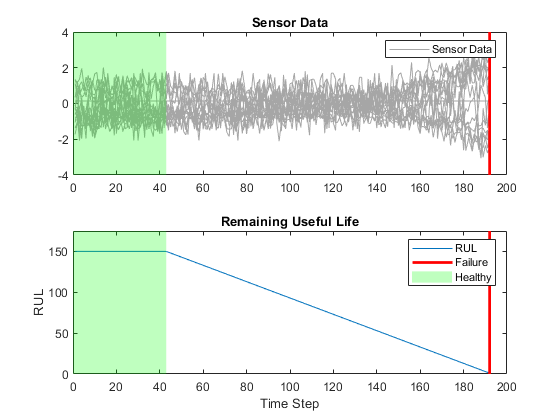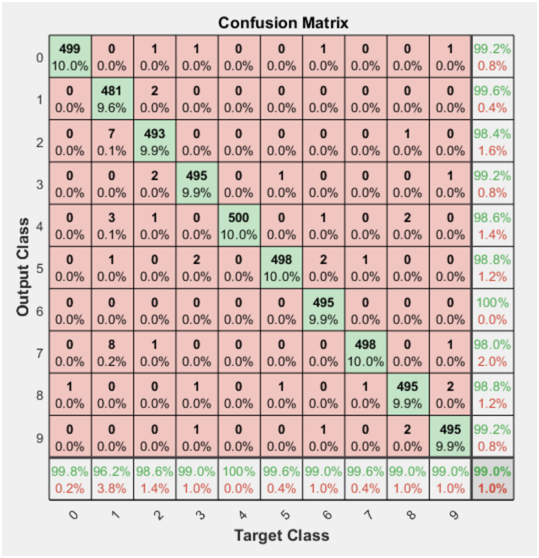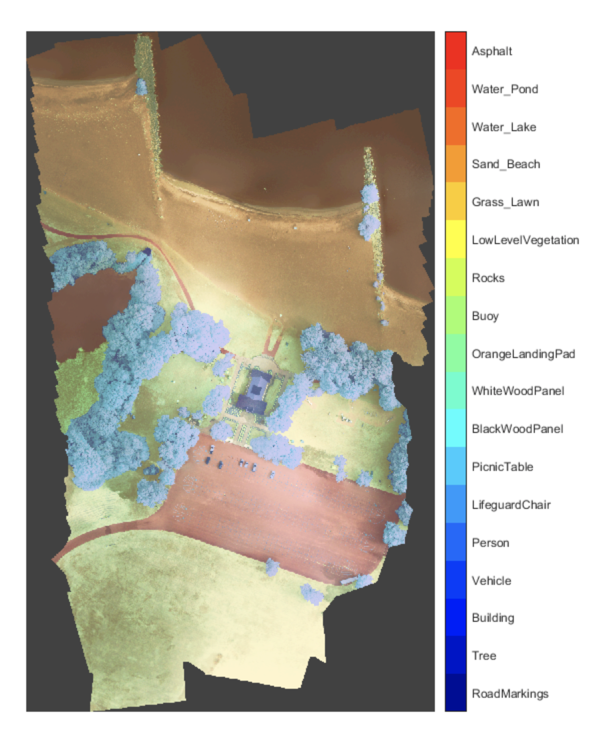New Deep Learning Features in R2018a

MathWorks shipped our R2018a release last month. As usual (lately, at least), there are many new capabilities related to deep learning. I showed one new capability, visualizing activations in DAG networks, in my 26-March-2018 post.

In this post, I'll summarize the other new capabilities. I'll focus mostly on what's in the Neural Network Toolbox, with also some mention of the Image Processing Toolbox and the Parallel Computing Toolbox.

Regression problems, bidirectional layers with LSTM networks

The doc example "Sequence-to-Sequence Regression Using Deep Learning" shows the estimation of engine's remaining useful life (RUL), formulated as a regression problem using an LSTM network. The new function bilstmLayer creates an RNN layer that can learn bidirectional long-term dependencies between time steps.New solver options

When you train a network, now you can select the Adams solver or the RMSProp solver. You can also introduce gradient clipping, which can help keep the training stable in the face of rapid increase in gradients.

Plot confusion matrix

Use the new plotconfusion function to show what's happening with your categorical classifications.The rows correspond to the predicted class (Output Class) and the columns correspond to the true class (Target Class). The diagonal cells correspond to observations that are correctly classified. The off-diagonal cells correspond to incorrectly classified observations. Both the number of observations and the percentage of the total number of observations are shown in each cell.

The column on the far right of the plot shows the percentages of all the examples predicted to belong to each class that are correctly and incorrectly classified. These metrics are often called the precision (or positive predictive value) and false discovery rate, respectively. The row at the bottom of the plot shows the percentages of all the examples belonging to each class that are correctly and incorrectly classified. These metrics are often called the recall (or true positive rate) and false negative rate, respectively. The cell in the bottom right of the plot shows the overall accuracy.

Multispectral Images

The previous restriction on the number of channels in a convolutional neural network has been relaxed. That opens up the possibility of using deep learning with multispectral images. See the Image Processing Toolbox documentation example, "Semantic Segmentation of Multispectral Images Using Deep Learning."DAG editing

It's easier now to replace a layer in a LayerGraph object by using the new replaceLayer function, which is in the Neural Network Toolbox Importer for TensorFlow-Keras Models support package. This function updates layer connections automatically.

Speed up transfer learning by freezing weights

When you are using transfer learning with a pretrained convolutional neural network, you can now try to accelerate the training process by freezing the weights in the initial network layers. The "Transfer Learning Using GoogLeNet" documentation example shows you how.

Use multiple GPUs, locally or in the cloud

Neural networks are inherently parallel algorithms. You can take advantage of this parallelism by using Parallel Computing Toolbox to distribute training across multicore CPUs, graphical processing units (GPUs), and clusters of computers with multiple CPUs and GPUs.

Training deep networks is extremely computationally intensive and you can usually accelerate training by using a high performance GPU. If you do not have a suitable GPU, you can train on one or more CPU cores instead, or rent GPUs in the cloud. You can train a convolutional neural network on a single GPU or CPU, or on multiple GPUs or CPU cores, or in parallel on a cluster. Using GPU or any parallel option requires Parallel Computing Toolbox.

Application-oriented deep learning examples

There are many detailed documentation examples that illustrate the deep learning in various applications. Here are some of the new examples in R2018a:

Be sure to check out the Release Notes for other enhancements and changes that you might be interested in.

|